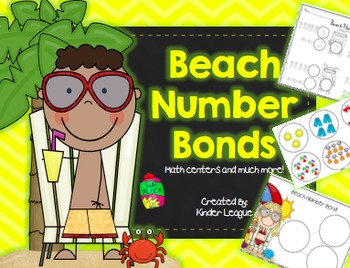# Beach Number Bonds by Kinder LeagueSubject
Resource Type
File Type

Zip

(3 MB|27 pages)
Standards
• Product Description
• Standards
Kinder League is here to save the day! We have created a hot, beach themed number bonds unit! In this unit you will get: math mats, number cards, and picture cards for whole group practice and/ or math centers. Number Bond Record sheets for students to record their results from their math mats, and number bond worksheets for independent practice and/ or Homework. This fun and amazing unit will inspire the students to master many of the CCSS!
This unit is complete with
8 math mats
6 pages of various number cards and picture cards for the math mats.
2 Number Bond Record Sheets
9 Independent Practice Worksheets/ Homework pages.
Fluently add and subtract within 5.
For any number from 1 to 9, find the number that makes 10 when added to the given number, e.g., by using objects or drawings, and record the answer with a drawing or equation.
Decompose numbers less than or equal to 10 into pairs in more than one way, e.g., by using objects or drawings, and record each decomposition by a drawing or equation (e.g., 5 = 2 + 3 and 5 = 4 + 1).
Solve addition and subtraction word problems, and add and subtract within 10, e.g., by using objects or drawings to represent the problem.
Represent addition and subtraction with objects, fingers, mental images, drawings, sounds (e.g., claps), acting out situations, verbal explanations, expressions, or equations.
Total Pages
27 pages
N/A
Teaching Duration
N/A
Report this Resource to TpT
Reported resources will be reviewed by our team. Report this resource to let us know if this resource violates TpT’s content guidelines.